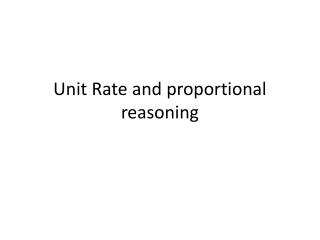DownloadDownload PresentationUnit Rate and proportional reasoning

# Unit Rate and proportional reasoning

Télécharger la présentation## Unit Rate and proportional reasoning

- - - - - - - - - - - - - - - - - - - - - - - - - - - E N D - - - - - - - - - - - - - - - - - - - - - - - - - - -
##### Presentation Transcript

1. Unit Rate and proportional reasoning

2. Warm up page 7 Write each ratio in simplest form.

3. Vocabulary Unit rate pg 8 • What is a rate? • A rate is a ratio that compare two quantities measured in different units. Suppose you read 233 words in two minutes. Your reading rate is

4. Unit rate pg 8 • What is a unit rate? • The rate for one unit of a given quantity. To find a unit rate, divide the first quantity by the second quantity. For your reading rate of , the unit rate is 116.5 words per minute.

5. Unite rate pg 8 • What is a unit price? • A unit rate that gives the cost per unit Example: Suppose the box of cereal mentioned earlier weighs 8 oz and has a unit price of \$0.31 per ounce. You want to find the total price of the box of cereal. 8 oz \$____ 1 oz \$0.31

6. Examples- unit rate pg 8 • A Box of cereal contain 8 serving and has a total of 36 grams of fat. Find the unit rate of grams of fat per serving.

7. Example- unit price pg 8 • The unit price for dog food is \$0.35/lb. How much is a 20lb bag?

8. Warm up pg 9 • Find the unit rate You earn \$33 dollars for 4 hours of work. How much did you make per hour? Sarah drove 203 mi to her cousins house. The drive took her 3 hours and 30 min. What was her average speed in miles per hour?

9. Check homework

10. Quiz page 9 under warm up • It took Anthony 5 hr 45 min to complete a 25 mi walkathon. What was his average speed in miles per hour? Round your answers to the nearest hundredth. • A 20lb bag of dog food cost \$21.50. A 30 lb bag of the same dog food cost \$32.90. Find each unit price. The determine the better buy.

11. Review pg 10 IAN • Problems 1-38 all page 249